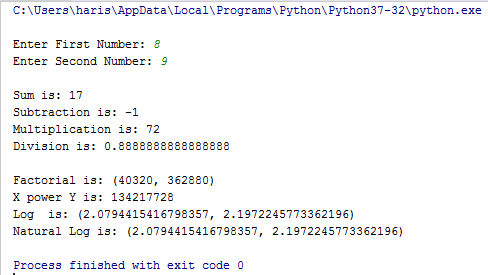# Python Learning Week 3 (Solution)

Create a class name​​ basic_calc​​ with following attributes and methods; Two integers (values are passed with instance creation) Different methods such as​​ addition, subtraction, division, multiplication​​

Create another class inherited from​​ basic_calc​​ named​​ s_calc​​ which should have the following additional methods;​​ Factorial, x_power_y,log, ln etc​​

• class child_class(parent_class): ​​

•  ​​ ​​ ​​ ​​ ​​​​ def __init__(self,x):​​

• ​​ #it will modify the _init_ function from parent class​​

• #additional methods can be defined​​

CODE:

import​​ math

class​​ basic_calc():
​​ ​​ ​​​​ def​​
__init__(self,​​ x,​​ y):
​​ ​​ ​​ ​​ ​​ ​​ ​​​​
self.x​​ =​​ x
​​ ​​ ​​ ​​ ​​ ​​ ​​​​
self.y​​ =​​ y

​​ ​​ ​​​​
def​​ sum(self):
​​ ​​ ​​ ​​ ​​ ​​ ​​​​ return​​
self.x​​ +​​ self.y

​​ ​​ ​​​​
def​​ subt(self):
​​ ​​ ​​ ​​ ​​ ​​ ​​​​ return​​
self.x​​ -​​ self.y

​​ ​​ ​​​​
def​​ mult(self):
​​ ​​ ​​ ​​ ​​ ​​ ​​​​ return​​
self.x​​ *​​ self.y

​​ ​​ ​​​​
def​​ div(self):
​​ ​​ ​​ ​​ ​​ ​​ ​​​​ return​​
self.x​​ /​​ self.y

class​​ sec_calc(basic_calc):
​​ ​​ ​​​​ def​​
__init__(self,​​ x,​​ y):
​​ ​​ ​​ ​​ ​​ ​​ ​​​​
self.x​​ =​​ x
​​ ​​ ​​ ​​ ​​ ​​ ​​​​
self.y​​ =​​ y

​​ ​​ ​​​​
def​​ factorial(self):
​​ ​​ ​​ ​​ ​​ ​​ ​​​​ return​​
(math.factorial(self.x),​​ math.factorial(self.y))

​​ ​​ ​​​​
def​​ x_power_y(self):
​​ ​​ ​​ ​​ ​​ ​​ ​​​​ return​​
pow(self.x,​​ self.y)

​​ ​​ ​​​​
def​​ Log(self):
​​ ​​ ​​ ​​ ​​ ​​ ​​​​ return​​
(math.log(self.x),​​ math.log(self.y))

​​ ​​ ​​​​ def​​ ln(self):
​​ ​​ ​​ ​​ ​​ ​​ ​​​​ return​​
(math.log(self.x),​​ math.log(self.y))

a​​ =​​ int(input("\nEnter First Number: "))
b=​​ int(input("Enter Second Number: "))
c​​ =​​ basic_calc(a,​​ b)
d​​ =​​ sec_calc(a,​​ b)
print(f'\nSum is:​​ {c.sum()}')
print(f'Subtraction is:​​ {c.subt()}')
print(f'Multiplication is:​​ {c.mult()}')
print(f'Division is:​​ {c.div()}')
print(f'\nFactorial is:​​ {d.factorial()}')
print(f'X power Y is:​​ {d.x_power_y()}')
print(f'Log ​​ is:​​ {d.Log()}')
print(f'Natural Log is:​​ {d.ln()}')

OUTPUT: